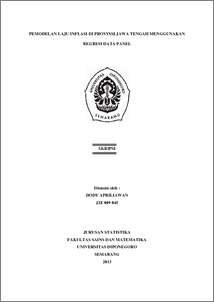# PEMODELAN LAJU INFLASI DI PROVINSI JAWA TENGAH MENGGUNAKAN REGRESI DATA PANEL

APRILIAWAN, DODY (2013) PEMODELAN LAJU INFLASI DI PROVINSI JAWA TENGAH MENGGUNAKAN REGRESI DATA PANEL. Undergraduate thesis, UNIVERSITAS DIPONEGORO.Preview
PDF
273Kb

## Abstract

Panel regression is a regression which is a combination of cross section and time series. To estimate the panel regression there are 3 approaches, the common effect model (CEM), the fixed effect model (FEM) and the random effect model (REM). In the CEM, the parameters were estimated using the Ordinary Least Square (OLS). In the FEM, the parameters estimated by OLS through the addition of dummy variables. At REM, error is assumed random and estimated by the method of Generalized Least Square (GLS). This study aims to analyze the factors that influence inflation in the Central Java province using panel regression. Based on test result of panel regression, the appropriate model is the CEM. The parameters of model are estimated by using OLS the cross section weights. The model show that the Consumer Price Index (CPI), Minimum Salary of City/Regency (MSCR) and the economic growth significantly effect on percentage of inflation in Central Java Province. Keywords: Common Effect Model, Fixed Effect Model, Panel Regression, Inflation in Central Java.

Item Type: Thesis (Undergraduate) H Social Sciences > HA Statistics Faculty of Science and Mathematics > Department of Statistics 42577 Mr Hasbi Yasin 03 Mar 2014 15:44 03 Mar 2014 15:44

Repository Staff Only: item control page